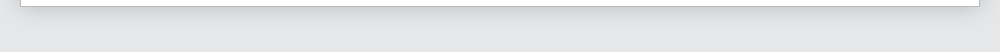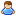Login Products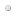SalesSupportDownloadsAbout
 Home » Technical Support » Elevate Web Builder Technical Support » Support Forums » Elevate Web Builder General » View Thread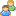View Thread

The following is the text of the current message along with any replies.
 Messages 1 to 2 of 2 total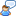find method and oncalculate row
 Fri, Dec 11 2015 9:12 AM Permanent Link Ronald Hi, It seems like having a Calculated column in a TDataset can interfere with the TDataSet.Find method. I use the find method in the following procedure: function TEdPlanningForm.FindAndPlan(Function:string):boolean; begin Result:=false; tbPlanning.InitFind; tbPlanning.Columns['function'].AsString:=Function; if tbPlanning.Find(false,true) then   begin   tbPlanning.Update;   tbPlanning.Columns['vak1'].AsInteger:=1;   tbPlanning.Save;   result:=true;   end; end; That works fine, until I use the following tbPlanning.CalculateRow function. Then the find method works correct the fisrt time I call it, but if I call it again it can not find the row. It seems like this CalculateRow interferes, what do I overlook? procedure TEdPlanningForm.tbPlanningCalculateRow(Sender: TObject; Column: TDataColumn); var t1, TempInt,CurValue :integer; begin TempInt:=0; for t1:=1 to 24 do   begin   CurValue:=tbPlanning.Columns['vak'+IntToStr(t1)].AsInteger;   if (CurValue<>0) and (CurValue<>PauzeTijdVak) then inc(TempInt);   end; tbPlanning.Columns['urenvandaag'].AsInteger:=TempInt; end; Thanks, Ronald Sat, Dec 12 2015 3:09 PM Permanent Link Tim Young [Elevate Software] Elevate Software, Inc.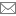timyoung@elevatesoft.com Ronald, << It seems like having a Calculated column in a TDataset can interfere with the TDataSet.Find method. >> Yeah, the OnCalculateRow event is firing when the dataset is in dsFind mode.  This is now fixed for 2.04, but here's the hot fix: WebData unit: procedure TDataRow.ValueChanged(Index: Integer); var   TempColumn: TDataColumn; begin   TempColumn:=FDataSet.Columns[Index];   if (not ((FDataSet.State=dsFind) or FDataSet.Calculating or TempColumn.Calculated)) then  <<< Change to this !!!!!      FDataSet.CalculateRow(TempColumn);   FDataSet.RowChanged(TempColumn); end; Tim Young Elevate Software www.elevatesoft.com